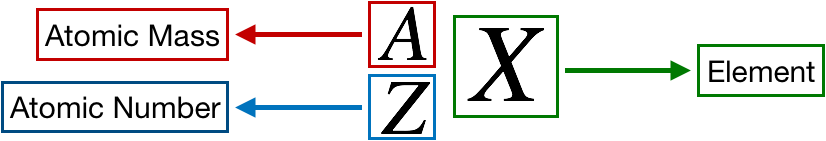# Problem: Calcium's atomic number is 20. It forms ions with 18 electrons. What is the electrical charge of a calcium ion?

###### FREE Expert Solution

We are being asked to determine the charge of a calcium ion with 18 electrons (atomic number of Calcium is 20).

An element or ion’s chemical formula is denoted as follows:99% (311 ratings)###### Problem Details

Calcium's atomic number is 20. It forms ions with 18 electrons. What is the electrical charge of a calcium ion?